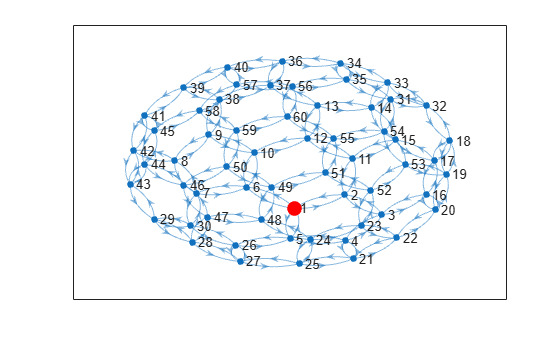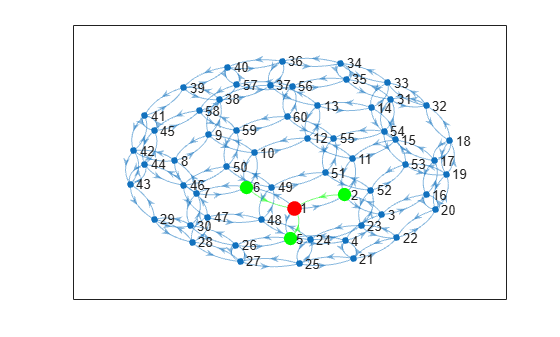inedges

Incoming edges to node

Description

example

eid = inedges(G,nodeID) returns the indices of all incoming edges to node nodeID in directed graph G.

example

[eid,nid] = inedges(G,nodeID) additionally returns the node IDs of the predecessor nodes connected to nodeID by the edges in eid.

Examples

collapse all

Create a multigraph with three nodes and four edges. Find the incoming edges of node 3.

G = digraph([1 1 1 2],[2 2 3 3]);
G.Edges
ans=4×1 table
EndNodes
________

1    2
1    2
1    3
2    3

eid = inedges(G,3)
eid = 2×1

3
4

G.Edges(eid,:)
ans=2×1 table
EndNodes
________

1    3
2    3

Plot a graph and highlight the incoming edges and predecessors of a selected node.

Create and plot a directed graph using the bucky adjacency matrix. Highlight node 1 for reference.

G = digraph(bucky);
p = plot(G);
highlight(p,1,'NodeColor','r','MarkerSize',10)Determine the incoming edges and predecessors of node 1. Highlight these nodes and edges.

[eid,nid] = inedges(G,1)
eid = 3×1

4
13
16

nid = 3×1

2
5
6

X = G.Edges(eid,:)
X=3×2 table
EndNodes    Weight
________    ______

2    1       1
5    1       1
6    1       1

highlight(p,nid,'NodeColor','g','MarkerSize',9)
highlight(p,'Edges',eid,'EdgeColor','g')Input Arguments

collapse all

Input graph, specified as a digraph object. Use digraph to create a directed graph object.

Example: G = digraph([1 2],[2 3])

Node identifier, specified as one of the values in this table.

ValueExample
Scalar node index1
Character vector node name'A'
String scalar node name"A"

Example: inedges(G,1)

Example: inedges(G,'A')

Output Arguments

collapse all

Edge indices, returned as a column vector. You can use the edge indices to index into the edges table of the graph with G.Edges(eid,:).

Node IDs of predecessors, returned as node indices if nodeID is numeric, or as node names if nodeID is a node name. Use findnode(G,nid) to convert node names into node indices. You can use node indices to index into the nodes table of the graph with G.Nodes(nid,:).

The node IDs in nid are the same as those returned by the predecessors function. However, if there are multiple incoming edges from the same node, this node is listed more than once in nid.

Tips

• By convention, for undirected graphs, all edges incident to a node are considered to be outgoing edges. Use outedges with undirected graphs.

• For graphs with multiple edges, inedges and predecessors can return arrays of different lengths, since there can be multiple incoming edges from some of the predecessors.